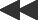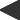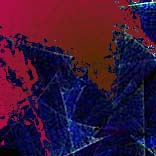basis: bifurcation pictorial representation of a population groth model. let P = new population, p = old population, r = growth rate the model is: P = p + r * fn(p) * [1 - fn(p)]. 3 parameters: filter cycles, seed population, and function.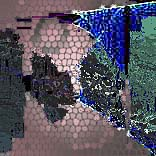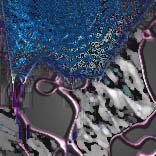The wonder of fractal geometry is that such complex forms can arise from such simple generating processes. A parallel surprise has emerged in the study of dynamical systems: that simple, deterministic equations can yield chaotic behavior, in which the system never settles down to a steady state or even a periodic loop. Often such systems behave normally up to a certain level of some controlling parameter, then go through a transition in which there are two possible solutions, then four, and finally a chaotic array of possibilities.